CLOSE

Recommanded Free YOUTUBE Lecture: <% selectedImage %>

## Monte Carlo Method

몬테카를로법은 어떤 문제를 수치 계산으로 풀지 않고 확률을 이용해서 푸는 방법입니다. 난수를 발생해서 푸는 거죠. 이번주 일요일 (2011년 10월 9일) 10월호 월간 뉴튼을 사러갔는데, "확률의 세계"라는 Newton 하이라이트 시리즈가 눈에 띄더군요. 수학을 싫어하기 때문에 그동안 눈길을 주지 않고 있었는데, 최근 통계쪽도 관심을 좀 가져볼까라는 생각을 가지려던 참이라 아무 생각없이 구입을 했습니다.

역시 저에겐 너무 어렵더군요.

그냥 샀으니 읽자라는 마음가짐으로 대략 훑어보는데, 몬테카를로 기법을 이용한 원주율 계산편이 눈에 들어오더군요. 신기하다거나 그랬다기 보다는 "이거 프로그램으로 테스트 해볼 수 있겠는데 !? gnuplot(:12)으로 시각화해볼 수 있겠다. 잼있겠다!"라는 생각이들더군요.

몬테카를로 기법을 이용해서 원주율을 계산하는 기본 개념은 간단합니다.

다음과 같이 반지름이 1인 원이 있다고 가정을 해보겠습니다. 좌표계산을 쉽게 하기 위해서 면적을 1/4로 재한했습니다. 그리고 이 원에 접선을 가지는 정사각형을 그립니다. 이 정사각형의 면적은 1이 되겠죠.이제 0~1 사이의 난수를 하나 발생시킵니다. 이 점은 2차원 평면안에 위치해야 하니까 {x,y} 좌표값을 가집니다. 이 점은 원안에 표시될 확률을 가질 겁니다. 이 확률을 계산하는 거죠. 난수로 발생한 점이 원안에 있는지는 피타고라스의 유명한 정리 "a^2+b^2=c^2"로 구하면 됩니다. 난수를 충분히 발생하면 원주율 값에 근접하는 값을 얻을 수 있을 겁니다.

수학적 방법으로 푸는 것과 비교하자면 명확한 답을 얻을 수는 없겠지만 대신 빠르게 매우 근접한 답을 얻을 수 있다는 장점이 있습니다. 소숫점 몇자리의 중요성 보다는 빠르게 대략적인 정보를 얻어야 할 때 사용할수 있겠네요.

gnuplot에 대한 내용은 GNU manual문서를 참고하시기 바랍니다.

## 프로그램 코드

코드는 perl로 만들었습니다.
```#!/usr/bin/perl
use strict;

my \$i = 0;
my \$x;
my \$y;
my \$inner = 0;
my \$outter = 0;
my \$randnum = \$ARGV;

print "#\tx\ty\tpie\n";
while (\$i < \$randnum)
{

if((\$x**2) + (\$y**2) <= \$radius **2)
{
\$inner++;
}
else
{
\$outter++;
}
\$i++;
my \$pie = (\$inner*4) / \$i;
print "\$i\t\$x\t\$y\t\$pie\n";
}
```
소숫점 계산을 피하기 위해서 원의 반지름을 1000으로 했습니다. 발생할 난수의 갯수는 프로그램 실행인자로 받아오게 했습니다. 확률값 즉 pie는 (\$inner * 4) / \$i로 했구요. 전체 점 중에서 원 내부에 찍힌 점의 비율입니다. 1/4 원이기 때문에 *4를 해줬습니다.

## gnuplot로 시각화 하기

### 난수 발생 횟수를 10으로 했을 때

프로그램의 실행 결과를 보겠습니다. 우선 10개의 점을 발생시켰습니다.
```\$ ./pie.pl 10
#       x       y       pie
1       81      243     4
2       163     186     4
3       760     874     2.66666666666667
4       993     697     2
5       930     279     2.4
6       368     90      2.66666666666667
7       37      281     2.85714285714286
8       511     754     3
9       91      310     3.11111111111111
10      578     957     2.8```
음.. pie가 2.8이 나왔네요. 3.14에서 한참이나 멀리 떨어져 있군요. 몬테카를로법은 큰 수가 준비되어야 쓸 수 있는 기법입니다.

이 결과를 파일로 저장하고 gnuplot로 시각화 했습니다. pie.pl의 실행 결과를 파일로 저장한다음 gnuplot를 호출했습니다. 저장한 파일의 이름은 plot.txt로, gnuplot 데이터 파일은 m10.dem으로 했습니다. 내용은 다음과 같습니다.
```# cat m10.dem
set parametric
set terminal png
set output "output.png"
set grid
set xrange[0:1000]
set yrange[0:1000]
plot "plot.txt" u 2:3 title "dot" lc rgb "blue",cos(t)*1000,sin(t)*1000 title "circle" lc rgb "red"```
• set terminal png : 결과는 png 이미지로 출력하게 했습니다.
• set output "output.png " : 이미지의 이름은 output.png
• set grid : 도표에 grid를 출력.
• xrange, yrange : x축과 x축의 범위 입니다.
출력 결과입니다. 1000 X 1000 면적에서 원이 차지하는 면적이 더 넓으니, 점이 원안에 위치할 확률이 더 높겠죠.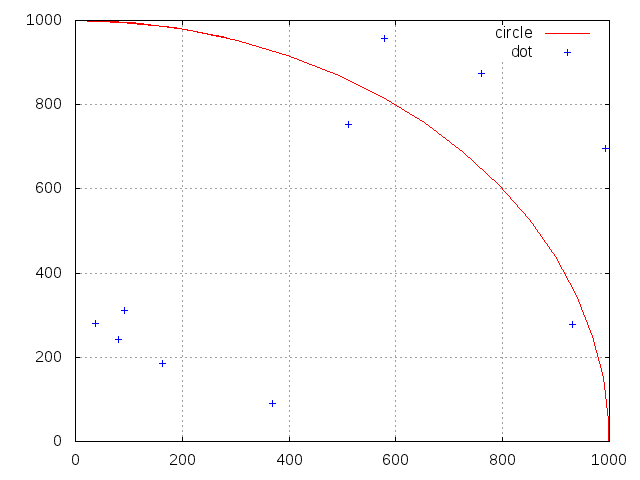보낸 사람 Linux

이제 확률을 계산해 보죠. 확률 계산을 위해서 사용한 gnuplot 데이터 파일의 이름은 y10.dem입니다.
```# cat y10.dem
set terminal png
set output "output2.png"
set grid
plot "plot.txt" u 4 with l, 3.14 title "Pie=3.14" linetype 5 ```

다음은 출력 결과입니다.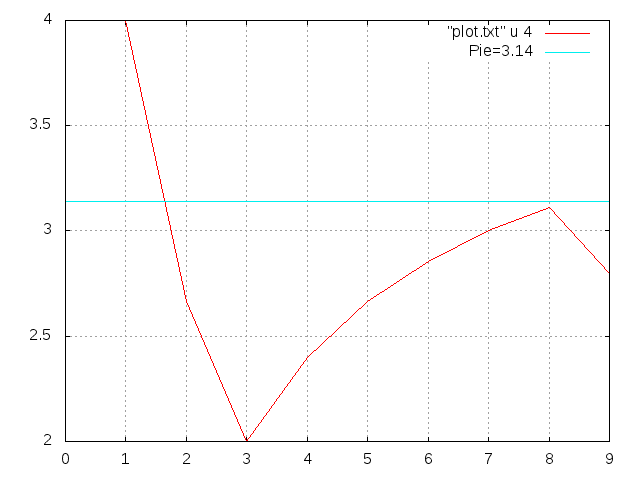보낸 사람 Linux
그닥 결과가 좋지 않군요. 이 정도로는 값이 의미하는 바가 뭔지 알수가 없습니다. 하지만 난수를 충분히 발생시킨다면, 결과가 달라지겠죠. 동전을 10번만 던져서는 확률을 계산하기 힘들지만, 100만번을 돌리면 1/2에 근접하는 정확한 값을 얻을 수 있는 것과 마찬가지죠.

### 난수 발생 횟수를 100으로 했을 때

난수 발생 횟수를 늘렸습니다.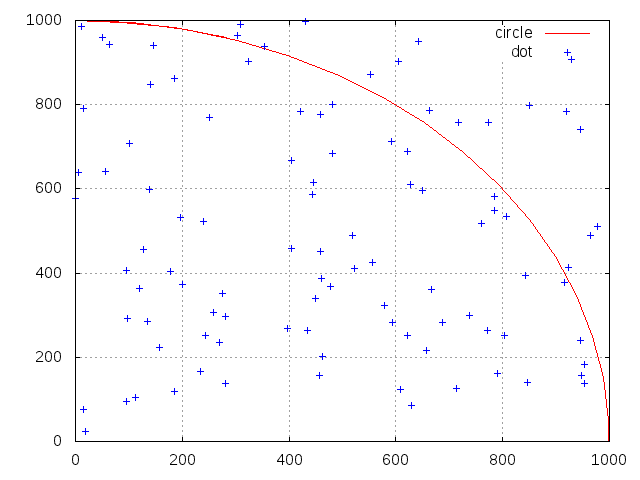보낸 사람 Linux

확률값 값입니다.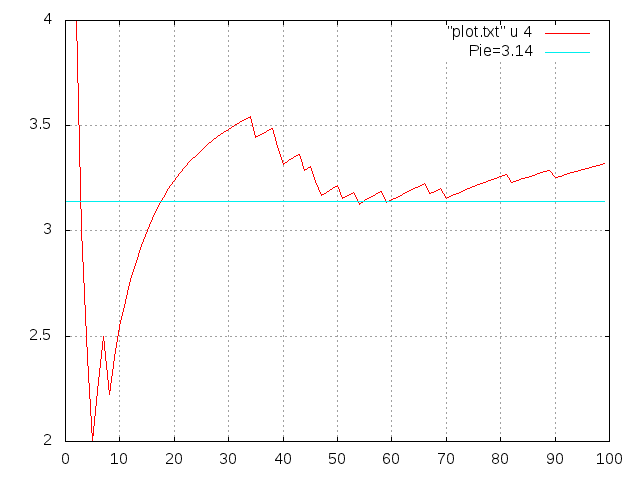보낸 사람 Linux
여전히 불규칙하기는 하지만 뭔가 pie값에 근접하는 추이를 볼 수 있죠 ?

### 난수 발생 횟수를 100000으로 했을 때

10만번 돌렸습니다. 돌렸더니.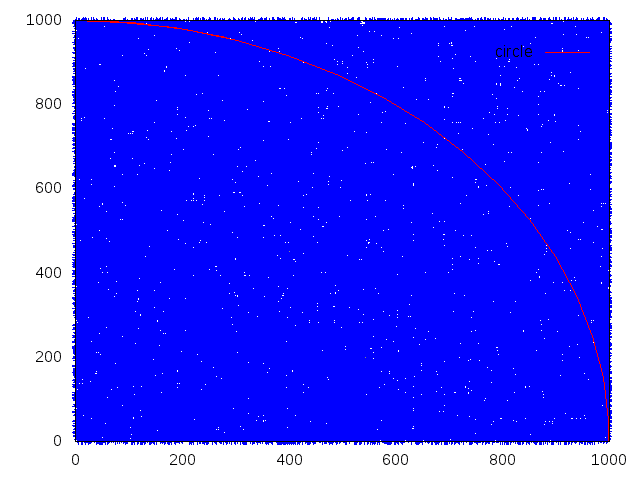보낸 사람 Linux
크 좌표를 거의 메우는 군요.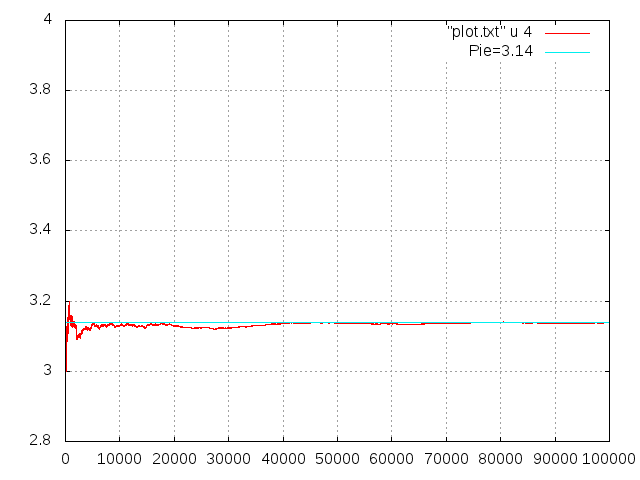보낸 사람 Linux
거의 3.14에 근접합니다. 실제 값은 3.13896으로 나왔습니다. 백만번 했더니, 3.145984 역시 난수가 많을 수록 계산값에 근접하게 되네요.

## 다른언어로

### C 코드

joinc 페이스북 그룹의 강두루님의 코드.
```#include <stdio.h>
#include <stdlib.h>
#include <math.h>

//10의 6승 까지 100,000번 시도 한다.
#define MAX_TRY (int) pow(10.0,8.0)

int xyGenerator(double *x, double *y)
{
*(x) = (double)rand()/32767;
*(y) = (double)rand()/32767;
return 1; //제대로
}

void acquisitionPI(int *Gn, int *Gi)
{
double x, y;
//10의 6승 까지 100,000번 시도 한다.

//int MAX_TRY = (int)pow(10.0,8.0);
for(*Gn = 1; (*Gn) <= MAX_TRY ;(*Gn)++)
{
xyGenerator(&x, &y);
//카운트 조건 식 : x제곱 + y제곱 =< 1
if((pow(x,2)+pow(y,2)) <= 1) (*Gi)++;
}
}

int main()
{
//변수 initialize
int Gi = 1, Gn = 1;
Gi = 1;

double result = 0.0;

acquisitionPI(&Gn, &Gi);

result = 4.0 * ((double) Gi / (double) Gn);

printf("파이 결과 값 : %f" , result);

return 0;
}```